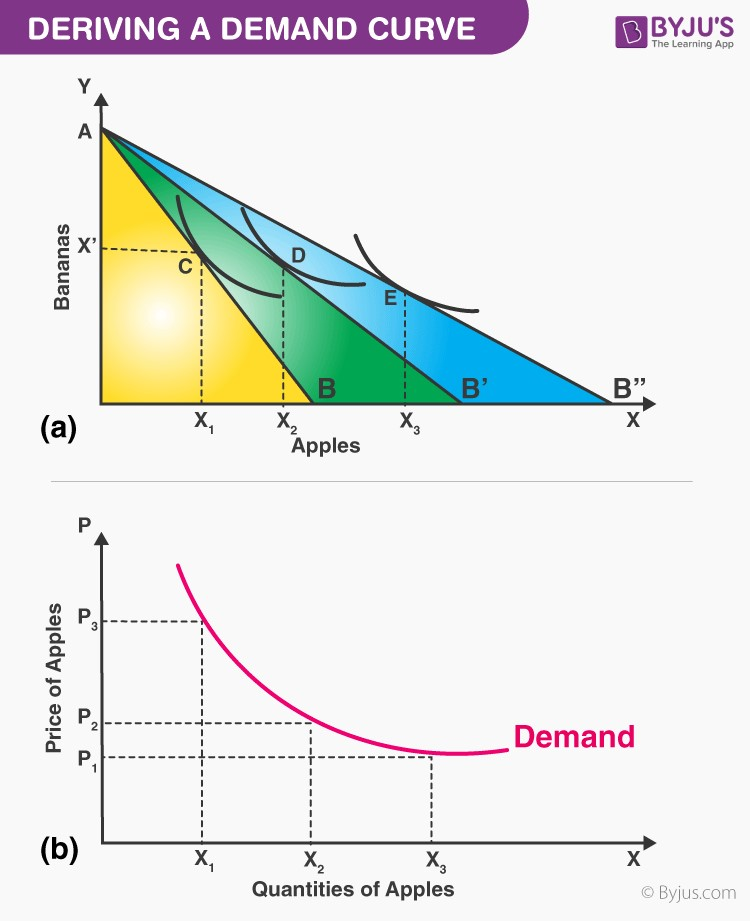# Deriving A Demand Curve From Indifference Curves

## How to derive an Individual’s Demand Curve from the Indifference Curve Analysis?

A demand curve depicts how much quantity of a commodity will be bought or demanded at various costs, presuming that the proclivity and tastes of a customer’s income and costs of all goods remain the same (constant).

The demand curve that depicts a clear association between the cost and quantity demanded can be obtained from the price utilisation curve of the indifference curve analysis.

According to the Marshallian utility analysis, the demand curve was derived on the presumption that utility was cardinally quantifiable and the marginal utility of money lasted constantly with the difference in price of the commodity. In the indifference curve analysis, the demand curve is derived without making these uncertain presuppositions.

Also read : Do you know the law of demand?

Suppose a person is consuming apples (X1)and bananas (X2), and that person’s income is N and the market prices of X1 and X2 are P’1 and P‘2 respectively. Figure (a) portrays their consumption equilibrium at point C, where they buy the X‘1 and X‘2 quantities of apples and bananas, respectively. In console (b) of the figure, we plot P‘1 against X‘1, which is the first point on the demand curve for X1.This is detailed and elucidated information on the concept of Deriving a Demand Curve from Indifference Curves and Budget Constraints. Stay tuned to BYJU’S to learn more.